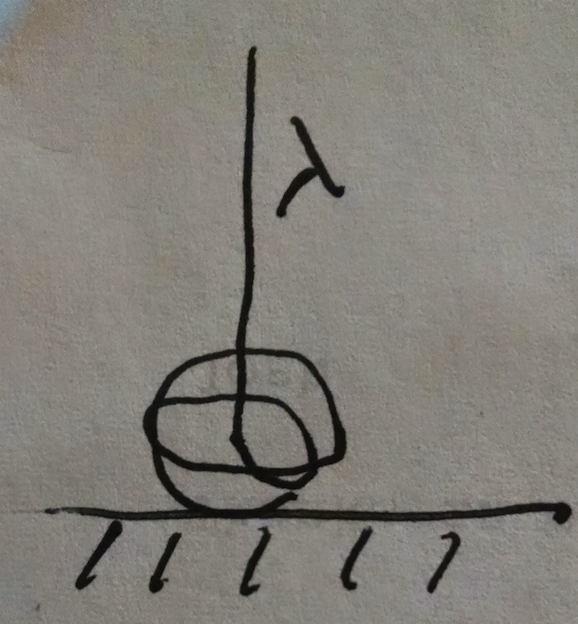Weight of a Falling ChainA 100 m rope of line density $\lambda=0.1$ kg/m is suspended vertically so that the bottom of the rope is just touching a scale. It is then released and falls onto the scale, which perceives a time dependent weight.

What is the reading on the scale in Newtons at the moment half the rope lies on the scale?

Assumptions and Details

• $g=9.8$ m/s$^{2}$.
×